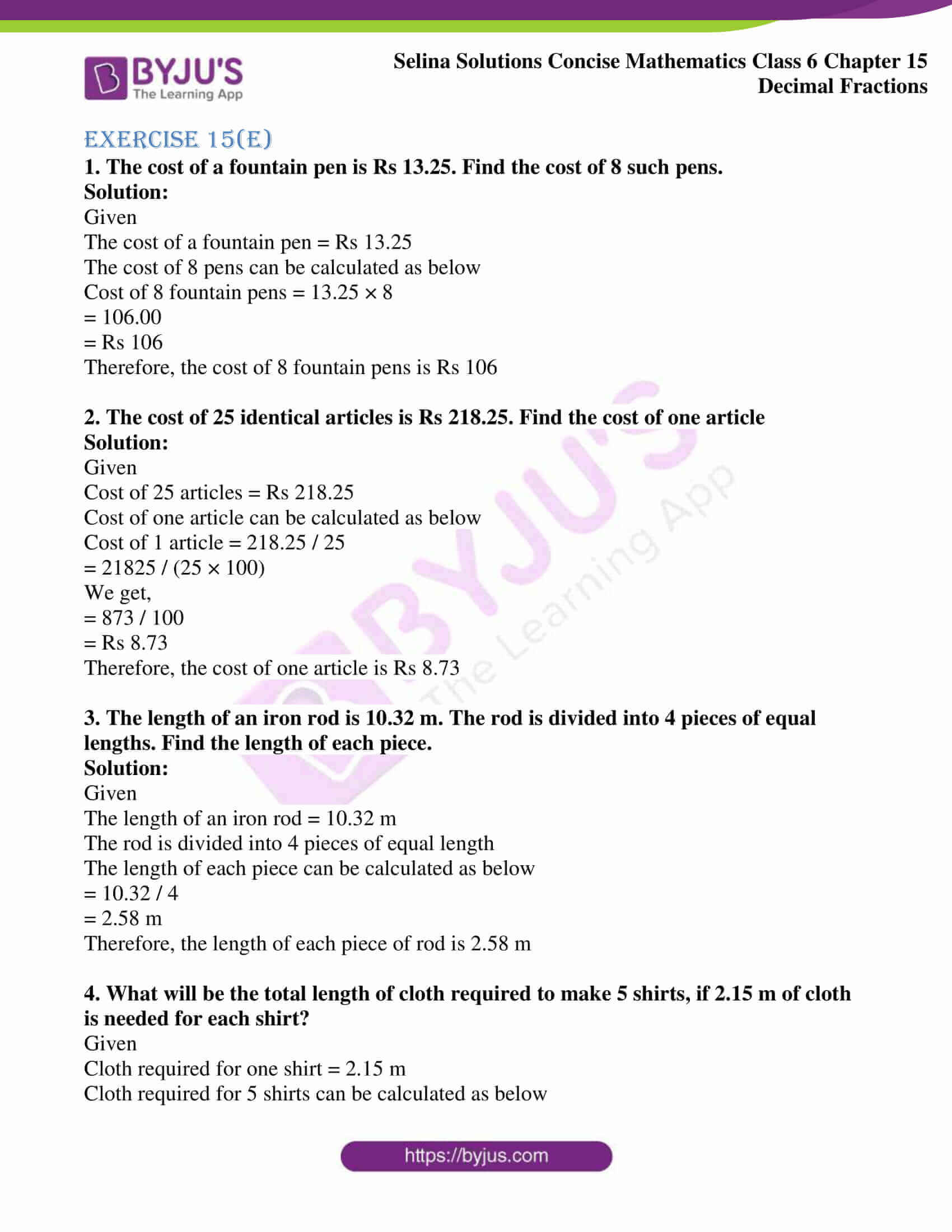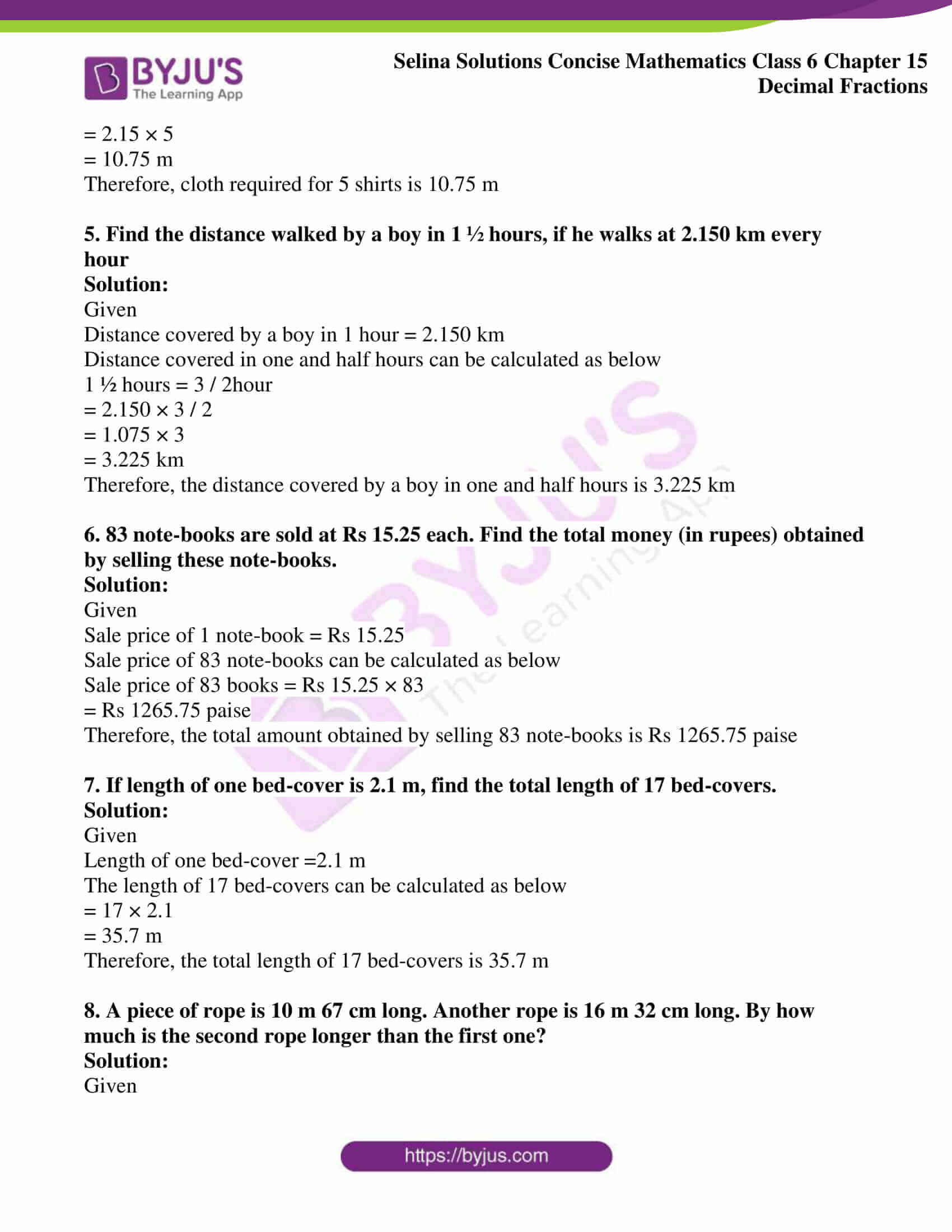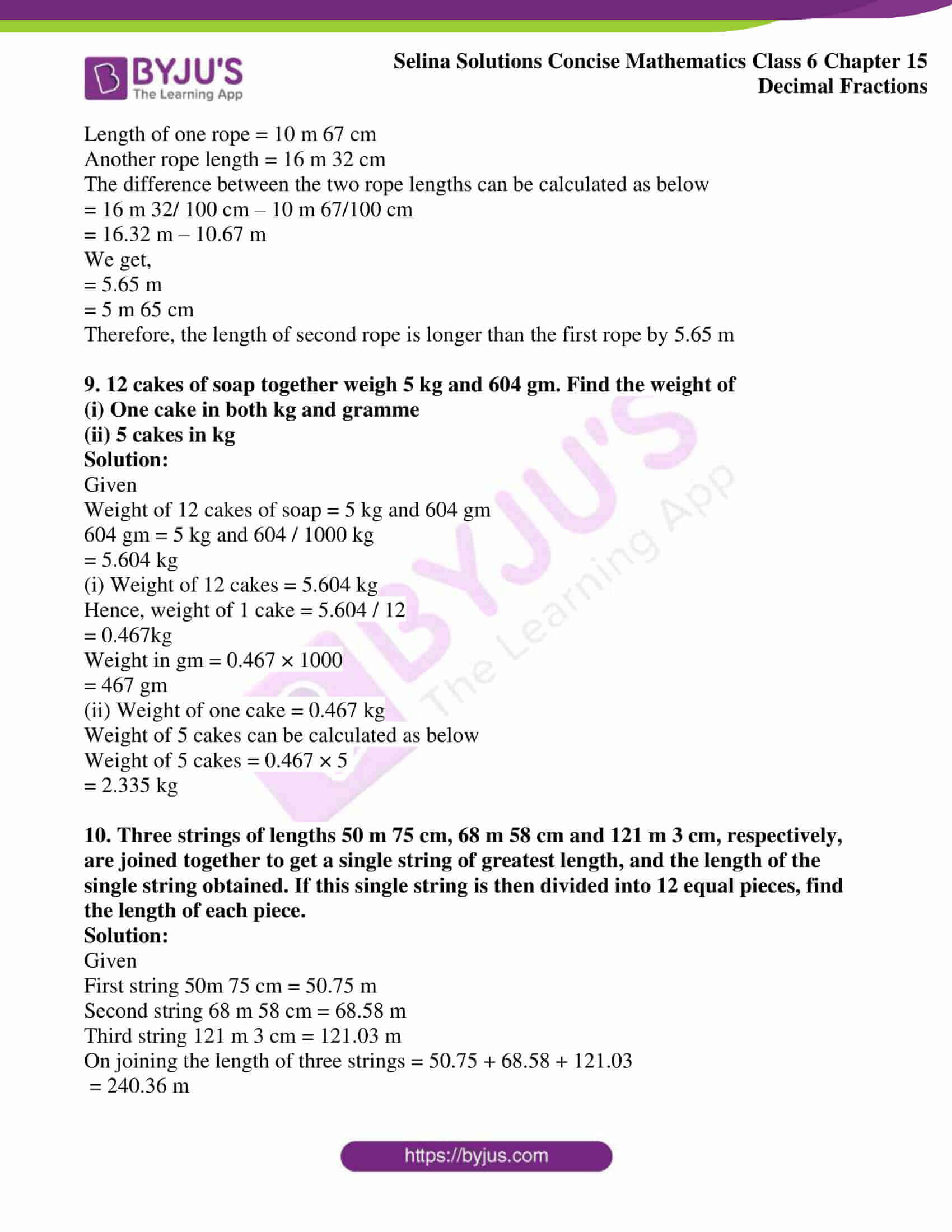# Selina Solutions Concise Mathematics Class 6 Chapter 15 Decimal Fractions Exercise 15(E)

Selina Solutions Concise Mathematics Class 6 Chapter 15 Decimal Fractions Exercise 15(E) are solved by teachers, having vast experience in the education industry. The main aim of providing solutions is to help students speed up their problem solving skills. The solutions have explanations in a descriptive manner to help students boost performance in the academic year. Regular practice of exercise wise problems using solutions PDF, help them to understand the concepts in-depth. Students can access Selina Solutions Concise Mathematics Class 6 Chapter 15 Decimal Fractions Exercise 15(E), PDF links which are given below

## Selina Solutions Concise Mathematics Class 6 Chapter 15: Decimal Fractions Exercise 15(E) Download PDF### Access other exercises of Selina Solutions Concise Mathematics Class 6 Chapter 15: Decimal Fractions

Exercise 15(A) Solutions

Exercise 15(B) Solutions

Exercise 15(C) Solutions

Exercise 15(D) Solutions

### Access Selina Solutions Concise Mathematics Class 6 Chapter 15: Decimal Fractions Exercise 15(E)

Exercise 15(E)

1. The cost of a fountain pen is Rs 13.25. Find the cost of 8 such pens.

Solution:

Given

The cost of a fountain pen = Rs 13.25

The cost of 8 pens can be calculated as below

Cost of 8 fountain pens = 13.25 × 8

= 106.00

= Rs 106

Therefore, the cost of 8 fountain pens is Rs 106

2. The cost of 25 identical articles is Rs 218.25. Find the cost of one article

Solution:

Given

Cost of 25 articles = Rs 218.25

Cost of one article can be calculated as below

Cost of 1 article = 218.25 / 25

= 21825 / (25 × 100)

We get,

= 873 / 100

= Rs 8.73

Therefore, the cost of one article is Rs 8.73

3. The length of an iron rod is 10.32 m. The rod is divided into 4 pieces of equal lengths. Find the length of each piece.

Solution:

Given

The length of an iron rod = 10.32 m

The rod is divided into 4 pieces of equal length

The length of each piece can be calculated as below

= 10.32 / 4

= 2.58 m

Therefore, the length of each piece of rod is 2.58 m

4. What will be the total length of cloth required to make 5 shirts, if 2.15 m of cloth is needed for each shirt?

Given

Cloth required for one shirt = 2.15 m

Cloth required for 5 shirts can be calculated as below

= 2.15 × 5

= 10.75 m

Therefore, cloth required for 5 shirts is 10.75 m

5. Find the distance walked by a boy in 1 ½ hours, if he walks at 2.150 km every hour

Solution:

Given

Distance covered by a boy in 1 hour = 2.150 km

Distance covered in one and half hours can be calculated as below

1 ½ hours = 3 / 2hour

= 2.150 × 3 / 2

= 1.075 × 3

= 3.225 km

Therefore, the distance covered by a boy in one and half hours is 3.225 km

6. 83 note-books are sold at Rs 15.25 each. Find the total money (in rupees) obtained by selling these note-books.

Solution:

Given

Sale price of 1 note-book = Rs 15.25

Sale price of 83 note-books can be calculated as below

Sale price of 83 books = Rs 15.25 × 83

= Rs 1265.75 paise

Therefore, the total amount obtained by selling 83 note-books is Rs 1265.75 paise

7. If length of one bed-cover is 2.1 m, find the total length of 17 bed-covers.

Solution:

Given

Length of one bed-cover =2.1 m

The length of 17 bed-covers can be calculated as below

= 17 × 2.1

= 35.7 m

Therefore, the total length of 17 bed-covers is 35.7 m

8. A piece of rope is 10 m 67 cm long. Another rope is 16 m 32 cm long. By how much is the second rope longer than the first one?

Solution:

Given

Length of one rope = 10 m 67 cm

Another rope length = 16 m 32 cm

The difference between the two rope lengths can be calculated as below

= 16 m 32/ 100 cm – 10 m 67/100 cm

= 16.32 m – 10.67 m

We get,

= 5.65 m

= 5 m 65 cm

Therefore, the length of second rope is longer than the first rope by 5.65 m

9. 12 cakes of soap together weigh 5 kg and 604 gm. Find the weight of

(i) One cake in both kg and gramme

(ii) 5 cakes in kg

Solution:

Given

Weight of 12 cakes of soap = 5 kg and 604 gm

604 gm = 5 kg and 604 / 1000 kg

= 5.604 kg

(i) Weight of 12 cakes = 5.604 kg

Hence, weight of 1 cake = 5.604 / 12

= 0.467kg

Weight in gm = 0.467 × 1000

= 467 gm

(ii) Weight of one cake = 0.467 kg

Weight of 5 cakes can be calculated as below

Weight of 5 cakes = 0.467 × 5

= 2.335 kg

10. Three strings of lengths 50 m 75 cm, 68 m 58 cm and 121 m 3 cm, respectively, are joined together to get a single string of greatest length, and the length of the single string obtained. If this single string is then divided into 12 equal pieces, find the length of each piece.

Solution:

Given

First string 50m 75 cm = 50.75 m

Second string 68 m 58 cm = 68.58 m

Third string 121 m 3 cm = 121.03 m

On joining the length of three strings = 50.75 + 68.58 + 121.03

= 240.36 m

Now,

One string = 240.36 m

Dividing the strings into 12 parts = 240.36 / 12

= 20.03 m

Therefore, the length of each piece is 20.03 m